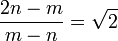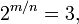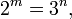# Irrational number

(Redirected from Rational number)

In mathematics, an irrational number is any real number that is not a rational number, i.e. it cannot be expressed as a fraction m / n in which m and n are integers.

##  Occurrence in geometry

Irrational numbers first entered the thinking of ancient Greek mathematicians because they arise in geometry. One of the simplest examples is the ratio of the length of the diagonal of a square to the length of the side of the square. By the Pythagorean theorem, that length of the diagonal is$\scriptstyle\sqrt{2}$ times the length of the side. It is possible to demonstrate that$\scriptstyle\sqrt{2}$ is an irrational number.

###  Proofs that √2 is irrational

Because of the negative nature of the definition of the concept of irrational number (an irrational number is a number that does not have a particular property), any proof that$\scriptstyle\sqrt{2}$ is an irrational number must be by reductio ad absurdum: assume it is rational and deduce contradictory conclusions.

####  First proof

If$\scriptstyle\sqrt{2}$ is rational, it can be expressed as a fraction m / n in lowest terms. Since the fraction m / n is in lowest terms, the numerator m and the denominator n are not both even. Since$\sqrt{2} = \frac{m}{n},$

it follows that$2n^2 = m^2.\,$

Since 2n2 is an even number, m2 is an even number. If m were odd, then m2 would be odd, so m must be even. Consequently m2 must be a multiple of 4. Therefore 2n2 must be a multiple of 4, and so n2 must be even. Then the same reasoning that told us m must be even now tells us that n must be even. But this contradicts our earlier conclusion that m and n are not both even.

####  Second proof

If$\scriptstyle\sqrt{2}$ is rational, it can be expressed as a fraction m / n in lowest terms. The numerator and denominator may be taken to be positive, since we can make them so if necessary by multiplying both by −1. A bit of algebra shows that if m and n are positive numbers and$\frac{m}{n} = \sqrt{2},$

then$\frac{2n - m}{m - n} = \sqrt{2}$

and 2n − m and m − n are smaller positive numbers than m and n respectively. This contradicts the earlier conclusion that the fraction m / n is in lowest terms.

##  π (pi)

A frequently cited example of an irrational number occurring in geometry is π = 3.14159..., the ratio of a circle's circumference to its diameter, or of the circle's area to the area of the square on its radius. This number is frequently approximated by 22/7 = 3.142857142857... But 22/7 is a rational number, so this cannot be exact.

Although the ancient Greeks worked with the number π, and in particular Archimedes showed that$3 + \frac{10}{71} < \pi < 3 + \frac{1}{7},$

no one at that time knew whether π is rational or irrational. That is a considerably more difficult problem than showing that √2 is irrational. It was not done until the 18th century. In the 20th century, a proof that π is irrational that requires no knowledge beyond integral calculus was discovered.

##  Certain logarithms

The numbers most easily proved to be irrational are certain logarithms. Suppose log2 3 is rational. That implies that it can be expressed as a fraction m / n, where m and n are integers. Since log2 3 > 0, we may take m and n to be positive, again if necessary by multiplying both the numerator and the denominator by −1. If$\log_2 3 = \frac{m}{n},$

then$2^{m/n} = 3,\,$

and hence$2^m = 3^n,\,$

but that is impossible because 2m is even and 3n is odd.Some content on this page may previously have appeared on Citizendium.